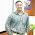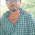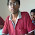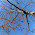# Pharma Engineering

An Engineer's Choice

• ## Monday, 2 May 2016

Great day viewers.....!!

Today i wish to have a brief about Motor selection for an agitator, this is quite important topic and before that you need to know how to select an agitator, and right now i'm not interested to demonstrate it as it will divert the topic from this point,

Getting into point, we should be well aware of the basic terminology for this topic, Like Equivalent Diameter, Bulk Velocity, Pumping Rate, Scale of Agitation, Power number, Reynolds Number.

Now, I gonna Elaborate you one by one, upto my knowledge.

Equivalent Dia : The defining of Equivalent Dia generally depends on the Pressure loss, Equivalent dia is nothing but just the Diameter of our reactor vessel diameter that gives the same pressure loss as an equivalent diameter of a rectangular vessel.

In other simple words, it means that the liquid level inside the vessel is equal to the vessel Dia, ad based on this only the Batch Sizing calculations were done.

Bulk Velocity/Pumping Velocity : The Velocity at which the Bulk mass in the vessel mixes/agitates around the shaft after gaining the momentum provided by the Agitator, this will have an high impact on Reaction and Work ups done in day to day production.

Pumping Rate :  The rate at which the Bulk mass gets Pumped inside the Stirred tank, this is partially depends on the HotSpots of the Stirred Tank and the utility in the Jacket of the stirred tank.

Scale of Agitation : Scale of Agitation gives the Intensity of Agitation or Mixing, Generally the Scale of Agitation will be in range of 1 to 10.

Scale of Agitation Range Values and their Significance over Agitation :

If Scale of Agitation is 1, then the effect of agitation is Mild, and this could be affordable when the operation is a crystallisation, that too if its a supersaturated Crystallisation.

If Scale of Agitation is 3, then the effect of agitation is Normal, this is most probably used for the Dryer's.

If Scale of Agitation is 6, then the effect of agitation is Vigorous, this means the mass that need to be stirred is somewhat denser but the bulk density will be not much.

If Scale of Agitation is 10, then the effect of agitation is Violent, this is applicable for the mass where there is need for extraction of product in between layers, and also the settling time during this extraction should be higher than regular cases.

Power Number : This number signifies the effect of Power consumed over the agitation, in other basic words it is ratio of resistance force to inertial force, it is dimensionless.

Reynolds Number : This number defines the fluid flow, so here it indicates the flow is whether laminar or turbulent.

So, now you were ready for the Show. I'll start it now,

Things you need to know is the Batch Volume ( V ), Type of Agitator, Agitator to vessel dia ratio ( D/T ), Scale of Agitation, Fluid Density ( rho ) and Fluid viscosity ( myu ).

Equate Batch Volume to the basic equation pi/4 x D x D x D., by replacing the Vessel Dia with Equivalent Dia, i.e.,

V = ( pi/4 ) x Teq x Teq x Teq

Then, Teq  = CubthRoot ( 4 x V/pi )

Agitator Dia ( D ) = D/T x Teq.

Bulk Velocity ( Vc )  =  6 x Scale of Agitation ft/min.

= 0.03048 x Scale of Agitation m/sec.

Pumping Rate ( Q ) = Vc. ( pi x Teq x Teq )/4        Cu.m/sec

Based on the Agitator, we need to consider the Flow number, find from below table.

From the Flow Number we need to determine the RPM, as shown below,

Nq = Q / ( N x D x D x D),

Agitator Speed , N =Q / ( Nq x D x D x D ),

From this Agitator Speed, calculate the Reynold's Number,

Nre = D x D x N x rho / myu.

rho - Density,  myu - Viscosity.

Now after Calculating the Reynolds number, we can get the Power Number from the Agitator Curves, as follows,

From the estimated Power number ( Np ), We can Calculate the Power required.

Power required ( P ) = Np x rho x N^3 x D^5.

You will be getting Power P in KW.

If you can understand all these say cheers, i've done my job, explaining it clearly, if you have any queries please feel free to ask me,

All Comments were Appreciated.Hi! I am Ajay Kumar Kalva, Currently serving as the CEO of this site, a tech geek by passion, and a chemical process engineer by profession, i'm interested in writing articles regarding technology, hacking and pharma technology.
Follow Me on Twitter AjaySpectator & Computer Innovations

1.Equate Batch Volume to the basic equation pi/4 x D x D x D., by replacing the Vessel Dia with Equivalent Dia, i.e.,

V = ( pi/4 ) x Teq x Teq x Teq .

pls. clarify what is D,Teq in above.
Secondly in attached excel sheet if we will take water in batch reactor then vicosity =1 cp ,then reynold's no. will be very high(in lakhs), then what will be the power no. in case of Pitch blade turbine & anchor .

1.Here D is the Vessel dia, Teq is the equivalent dia,
Equivalent dia is nothing but just a assumption, for example if you can transform it into a rectangular which will he having same capacity of holding pressure, then the formed circle's dia is the equivalent dia of the vessel,

and what ever the reynolds number you may get don't get shock, it will be correct only

2.Sir i have a vertical round ms tank of size 6feet diameter and 8feet height with wall thickness 5mm for lubricànt blending suggest me the motor and gear box details with agitator design my contact number 9826186101

3.Dear ,

Lubricants were of high viscosity, so i prefer anchor as agitator, As you have mentioned 6 ft dia, the anchor dia should be 70-80% of tank dia, so Anchor dia should be ~1.3 m,
By the dimensions that you have provided me, the volume is coming around 6.4 KL,
as you are going to handle viscous liquid, go with 60% of safe working volume, so it will be 3.8 KL,
Anchor will have an RPM of 48,
For anchor, Power no. will be 3-5[usually power no will vary based on reynolds no.]

So now the power consumption will be 8554.6 J/S = 8554.6 Watts = 8.6 KW.
For calculation of power i've taken density as 1500 Kg/Cu.m.

for power calculation, refer the below link:
https://pharmacalc.blogspot.com/2016/05/motor-selection-for-agitator.html

And coming to Motor capacity, we have to consider approx. 30% excess to the required capacity, so the motor capacity will be 8.6 * 1.3 = 11.18 KW ~11.5 KW = 15.4 HP
~16 HP.

Usually a motor will have an RPM of ~1475, so to match the anchor RPM to 48,
Gear box should have an ratio of 1475 / 48 = 30.75.

Still any queries feel free to comment / contact me,

Best Regards,
AJAY K

4.Dear Ajay Ji,

Good Morning,

I am designing anchor type agitator. Tank Diameter is 2525 mm, Tank height is 2250 mm, Liquid Viscosity is 3000 Cp, Density is 1500 Kg/m3, Blade diameter is 2480 mm, Blade width is 50 mm, Blade thickness is 10 mm, Hight is 16oo mm. Pl advise me to ho to calculate the Required power for agitator.
A.P. Singh
Kanpur
M-9450752035

5.Hiii,

High dense and viscous liquid, So agitator should be anchor / ribbon gate / max blend / shear plough agitator. and the agitator selection is correct.
Everything is available, just application lacks here.
Anyhow below is for you,

P = Np x density x (RPS^3) x (Impeller Dia^5).

= 3 x 1500 x (48/60)^3 x (2.48^5) = 216143 J/Sec = 216.14 KW = ~290 HP.

** As the operational RPM is not mentioned, i've considered Power no. as 3 and RPM as 48.

Regards,
AJAY K

2.Hello Ajay,
I Have to increase the speed of agitator. ( Current detail is Agitator RPM=48, Motor RPM 1400, Agitator type= Anchor with paddle type, Reactor SSR 250 Lit.). We need to increase the RPM up to 75-80 with same agitator. Is it possible to increase the agitator RPM with increasing Motor RPM.

1.Dear ,

First of all glad to hear from you,
As per the current details, your gear box ration is 29.2,
48 RPM for anchor means the agitation will be some what vigorous,
and you are telling that you need to increase the RPM to 75/80, it is not appropriate to enhance the RPM upto 75/80.
Anyway if you want to increase the RPM, then better change the gearbox, with ration 17.5. you haven't mentioned anything about motor power. as per my knowledge if 75/80 RPM needed, its better to go with a 5 KW motor.

Regards,
AJAY K

3.Dear ajay

Above explanation is quite useful.Now we can also discuss design on NRE>1 LAKH.
Also calculation of flat blade turbine power number.

1.Dear Anshu,

you can take power number as 3.60 for a FBT, and the remaining calculation will be same.
once refer this site: https://www.chemicalonline.com/doc/flat-blade-turbine-fbt-0001
Regards,
AJAY K.

4.Dear Ajay Sir,
If you please tell me when(e,g in the time of crystalization, slurrification, suspension) axial mixing and when radial mixing required?

1.Dear Pratim,

During reaction it is preferred to go with radial flow, and while extractions / washing better to go with axial flow,
During crystalization suggested to go with axial mixing with low RPM, while slurrification move with radial flow.

Regards,
AJAY K

5.Hello Sir,

I am working at Lupin Ltd..

In one of our product, the isolation is done in centrifuge..The CF is SS 48".. The issue is the wet cake becomes hard and during unloading we need to use scrapper which causes the CF bag damage..

Is there any means available by which we can avoid the usage of scrapper..

The solvent is chloroform..

1.Dear Rohit,

As we know that its not a safe practice to unload chloroform rich wet material, and if you cant use a scrapper, try it out with a scoop, use an anti-static nylon double bag or else go ahead with bottom discharge centrifuge.

Regards,
AJAY K

6.hi, I need agitator for rectangle tank with volume of 150 liter , can u suggest ?

1.Dear,

go ahead with a gate agitator, enhance the sweep dia to 80% of the tank dia.

Comment with you name next time,

Regards,
AJAY K

7.what to assume D/T ratio?

1.Dear Sri,

For better results don't assume, D / T = Agitator Dia / Tank Dia, which you can get from GA drawings.

Regards,
AJAY K

8.Dear Ajay,
Upon solving, i arrived at higher value for power. Then i checked with excel formula, the answer is multiplied by 0.001341. What does this number for? Thank you

9.Dear Sir,
Got it already, its a conversion of watts to hp. Learned a lot on this. thank you so much for this helpful information.

1.Gud work Andy, these days i'm little busy in my profession, so i may be quite unavailable.

So i cant reply back to all.

10.Does the power increase if we increase the tier of impellers, for example two tier PBT,with 50% sweep dia
Vessel volume is 14kl

1.Dear ,

Based on impellers the power no. will vary, so based on that the power consumption will also vary.

Regards,
AJAY K

11.umesh kumar
pleare Sir! help me
dia 1.2m,height 1m liquid tooth paste anchor RPM is 48 . so please give me detail full explaination how to select power no,viscosity and density.Also explain how to select power no by the Reynold no and power of motor in hp

1.Dear Umesh,

Power required for mixing is calculated based on formula, Np x rho x (N^3) x (D^5).
Density(rho) of the toothpaste is a known property to u,
N is the RPS, so it is 48/60, D already you have mentioned as 1.2 m,
All you have to know is power no., and as per my suggestion don't take it based on thumb,
it shall be taken from agitator curves based on the reynolds no., and i'll explain you now,
Usually reynolds no. is a ration of inertial force to viscous force.
If viscous force is high, then the power consumption will be high
Re = N x rho x (D^2)/myu.
= (48/60) x 1.51 x (1.2^2)*1000/100000 = 17.39.
For reynolds no. of 17.39, the power number will be in range of 8-9.

Now calculate power, P = 9 x 1510 x (48/60^3)*(1.2^5) = 17313.93 watts = 17.31 KW
= 23.2 HP.
You can find the agitator curves here:
in the attached link, curve 1 represents anchor.

If any queries, feel free to comment/contact.

Regards,
AJAY K

12.Its great.
but ajay how we can find gear box / motor rpm ratio??

1.Dear ,

Usually motor will have an RPM of 1450 / 1475,
So, now if you want to select a gearbox for anchor,
the ratio shall be 1450/48 = 30.20
A gearbox whose frame size suits to the agitator coupling shall be selected with an ratio of ~30.20.

Hope it is clear,
Next time comment with you gud name plz.

Best Regards,
AJAY K

13.HI
I'm designing an agitator (Rushton turbine) for a tank of 167 m3, diameter of 5.21m and height of 7.82m. Following the calculations you did I got power of around 583kW for the agitator which seems really high. The viscosity of the fluid is 4.5cp and density is 1210kg/m3. Could you please let me know if the power value is reasonable. Thanks

Kind regards,
Numrah

1.Let me calculate,

Rushton 6 blade turbine usually will have dia of tanks 1/3rd.
Dia = (1/3) x 5.21 = 1.74 m,
let the occupancy be 80% = 167 x 0.8 = 133.6 m3
density be 1210 Kg/m3,
As its a rushton turbine, i'm considering an rpm of 100,

Power = 3 x 1210 x (100/60)^3 x (1.74^5) = 269637 watts = 269 KW = 360 HP.

Regards,
AJAY K

14.These calculations assume that the size of the mixing tank is known. Do you have any advice/ resources for calculating the required size (or residence time) of a mixing tank for good mixing?

1.Dear Brother,

Everywhere and everyone's desire is to attain good mixing only, but to attain those mixing patterns we need some inputs like the input RM's physical and chemical properties.
With those we'll be able to decide the scale of agitation to attain adequate mixing.
So requesting you to mention the case clearly, you can reach me at pharmacalc823@gmail.com.

Regards,
AJAY K

15.Dear Mr. Mr. Ajay,

Please suggest us capacity of the motor required for following details:
Tank Height : 1640mm, Tank Dia: 1040mm, Agitator/Blade type : Dispersing Homogenizing Blade, Viscosoity: 8000, Blade Dia : 450mm, Each batch : 85% filled tank, Motor directly coupled vertically downward, Agitator RPM = Motor RPM=1440. Please suggest motor capacity calculation.Your earliest response would be highly appreciated.

rgds

Akhilesh Gupta

1.Hii Akhilesh,

Power number for a homogenizing blade would be approx. 0.3, Density - 1000 Kg/m3(Considered), RPM - 1440, Dia of impeller - 0.45m.

Power = 0.3 x 1000 x (1440/60)^3 x 0.45^5 = 74649.6 Watts = 74.65 KW.

I've considered water density, as if its other solvent, it will vary.

Regards,
AJAY K

2.Dear Ajay,

Thanks for the support.

rgds

Akhilesh

16.regarding Power calculation for Homogenizing blade, vessel volume and viscosity are not considered for calculation. Eg. Vessel volume is 5 KL, viscosity 10000 CP, SG - 1.45, In this case what will be motor HP. It is twin shaft dispenser, There will be a slow speed scrapper also like Anchor blade. scrapper RPM would be 40.

1.Dear ,

Based on the viscosity of the reaction mass we will select the agitator and the power number is agitator specific. In the calculation we'll consider the power number, that means we'll indirectly considering the viscosity also(Not specifically).

Agitator dia depends on the vessel dia only. For anchor the dia will be 70-80% of vessel dia, for PBT dia will be 30-50% of tank dia, for RCI dia will be 50-70% of tank dia, etc...,

Power = 3.5 x 1450 x (40/60)^3 x (1.24^5) = 4401 watts = 4.4 KW = 5.9 HP ~6 HP

As you said that the agitator will have a scrapper blade, i've considered 95% of sweep. for a 5KL vessel the dia will be approx. 1.3 m. Power number is a dependent of reynolds number. Hence i've considered 3.5. Sometimes it may be quite higher, based on that the power will vary.

Any queries feel free to comment or mail me.

Regards,
AJAY K

17.If diameter of vessel not equal to height of liquid how can we calculate power requirements

1.Dear ,

Cant get your logic, can you please elaborate ?

Regards,
AJAY K

18.Sir
Suggest me require motor power for paddle type agitator along with gear box ratio . According to following parameters
(1) tank type - rectangular
(2) tank dimensions- 3m(h) × 1.2m×2.5m
(3) liquid density :- 1200kg/m3

1.Hii Ajay,

Thanks for the query.

Paddle agitator i'm considering around 1m,

Power required = Np x density x (RPM^3) x (dia^5) = 1.8 x 1200 x ((70/60)^3) x (1^5)

= 3430 watts = 3.43 KW = 4.6 HP.

Consider 85% efficiency of motor, required motor power = 5.4 HP.

** RPM and the agitator dia can be changed based on your requirement.

Best Regards,
AJAY K

2.Most of the times the motor RPM will be approx. 1500 (varies between 1450 - 1475).
Maintained RPM = 70, hence gearbox ratio = 1500/70 = 21.4. Hence the gearbox can be between 15-20.

19.Sir, I need type agitator & motor power for tank capacity 800m3
Dimension tank : L : 18m H : 5m W: 9m

20.Dear sir
Please degain the 7.5 kl motor and gear box capacity calcation brifely explan.

Regards
Narendra Paritala

1.Dear Narendra,

Motor power is calculated based on the power number,P = Np x density x (N^3) x (D^5).

D is impeller dia, N - RPS.

Gear box rating shall be calculated as , Motor RPM / Agitator RPM.

If its anchor, then the max RPM shall be 60. Gearbox ratio = 1475 / 60 = 24.58 ~25.

Regards,
AJAY K

21.SirHi! I am Ajay Kumar Kalva, Currently serving as the CEO of this site, a tech geek by passion, and a chemical process engineer by profession, i'm interested in writing articles regarding technology, hacking and pharma technology.